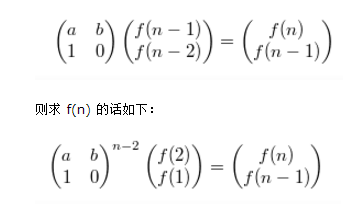# 前言

求一个数的n次方，朴素的算法就是直接for循环，一遍一遍的乘，a*a*a*a*a*a… …，O（N）的复杂度。此时，如果n很小的话，并没有什么影响。
但是当n非常大,n=10^18，O（N）也会超时，那么需要更快的算法，二分幂算法 和 快速幂算法。

# 二分幂

那么按照这个思路就能运用分治的思想,那么复杂度就有原来的O(n)，降低为O(lgn)。

long long int pow(int a,int n)//求a的n次幂
{
if (n==0)
return 1;
if (n==1)
return a;
long long int ans=pow(a,n/2);//从函数的功能区理解递归
ans*=ans;
if (n%2==1)
ans*=a;
return ans;
}

# 快速幂算法

A=a156=a10011100$a^{156}=a^{10011100}$
=a271+260+250+241+231+221+210+200$a^{2^7*1+2^6*0+2^5*0+2^4*1+2^3*1+2^2*1+2^1*0+2^0*0}$
=(a271)(a260)(a250)(a241)(a231)(a221)(a210)(a200)$(a^{2^7}*1 ) * (a^{2^6}*0) * (a^{2^5}*0) * (a^{2^4}*1) * (a^{2^3}*1) * (a^{2^2}*1) * (a^{2^1}*0) * (a^{2^0}*0)$

long long int fun( int a, int b )
{
long long int r = 1;
int base = a;
while( b != 0 )
{
if(b & 1)//判断奇偶性
{
r *= base;

}
base *= base; //注意：a^{2^7}=a^{2^6} * a^{2^6} ，而不是 a^{2^7}=a^{2^6} * a ，所以这是对的。
b /= 2;//与b=b>>1相同
}
return r;
}

# 矩阵快速幂算法# include<cstdio>
# include<cstring>
using namespace std;
#define NUM 50
int MAXN,n,mod;
struct Matrix//矩阵的类
{
int a[NUM][NUM];
void init()           //将其初始化为单位矩阵
{
memset(a,0,sizeof(a));
for(int i=0;i<MAXN;i++)
a[i][i]=1;
}
} A;
Matrix mul(Matrix a,Matrix b)  //(a*b)%mod  矩阵乘法
{
Matrix ans;
for(int i=0;i<MAXN;i++)
for(int j=0;j<MAXN;j++)
{
ans.a[i][j]=0;
for(int k=0;k<MAXN;k++)
ans.a[i][j]+=a.a[i][k]*b.a[k][j];
ans.a[i][j]%=mod;
}
return ans;
}

Matrix add(Matrix a,Matrix b)  //(a+b)%mod  //矩阵加法
{
int i,j,k;
Matrix ans;
for(i=0;i<MAXN;i++)
for(j=0;j<MAXN;j++)
{
ans.a[i][j]=a.a[i][j]+b.a[i][j];
ans.a[i][j]%=mod;
}
return ans;
}

Matrix pow(Matrix a,int n)    //(a^n)%mod  //矩阵快速幂
{
Matrix ans;
ans.init();
while(n)
{
if(n%2)//n&1
ans=mul(ans,a);
n/=2;
a=mul(a,a);
}
return ans;
}

Matrix sum(Matrix a,int n)  //(a+a^2+a^3....+a^n)%mod// 矩阵的幂和
{
int m;
Matrix ans,pre;
if(n==1)
return a;
m=n/2;
pre=sum(a,m);                      //[1,n/2]
ans=add(pre,mul(pre,pow(a,m)));   //ans=[1,n/2]+a^(n/2)*[1,n/2]
if(n&1)
ans=add(ans,pow(a,n));          //ans=ans+a^n
return ans;
}

void output(Matrix a)//输出矩阵
{
for(int i=0;i<MAXN;i++)
for(int j=0;j<MAXN;j++)
printf("%d%c",a.a[i][j],j==MAXN-1?'\n':' ');
}
int main()
{
freopen("in.txt","r",stdin);
Matrix ans;
scanf("%d%d%d",&MAXN,&n,&mod);
for(int i=0;i<MAXN;i++)
for(int j=0;j<MAXN;j++)
{
scanf("%d",&A.a[i][j]);
A.a[i][j]%=mod;
}
ans=sum(A,n);
output(ans);
return 0;
}  

# 快速乘

100110111010=1001101241+1001101231+1001101220+1001101211+1001101200$1001101 * 11010 = 1001101 * 2^{4} * 1 + 1001101 * 2^{3} * 1 + 1001101 * 2^{2} * 0 +1001101 * 2^{1} * 1 + 1001101 * 2^{0} * 0$

long long int fun(long long int a ,long long int b , long long int m)
{
int sum=0;
int k=1;
while(b)
{

if(b&1)
{
sum=(sum+a*k)%m;
}
k=(k*2)%m;
b=b/2;
}
}

1.多重背包问题
2.树状数组
3.状态压缩DP
……………还有很多。。。究其根本还是那句话：化连续为离散。。很多时候我们并不是为了解决一个问题而使用二进制，更多是时候是为了优化而使用它。所以如果你想让你的程序更加能适应大数据的情况，那么学习学习二进制及其算法思想将会对你有很大帮助。